Python 内存优化的方法白羽 2018-07-03 来源 ：网络 阅读 104 评论 0

Pytracemalloc

Python

>>> import sys

>>> sys.getsizeof(1)

24

>>> sys.getsizeof([])

72

>>> sys.getsizeof(())

56

>>> sys.getsizeof({})

280

>>> sys.getsizeof(True)

24

· Traceback where an object was allocated

· Statistics on allocated memory blocks per filename and per line number: total size, number and average size of allocated memory blocks

· Compute the differences between two snapshots to detect memory leaks

import tracemalloc

NUM_OF_ATTR =  10

NUM_OF_INSTANCE = 100

class Slots(object):

__slots__ = ['attr%s'%i for i in range(NUM_OF_ATTR)]

def __init__(self):

value_lst = (1.0, True, [], {}, ())

for i in range(NUM_OF_ATTR):

setattr(self, 'attr%s'%i, value_lst[i % len(value_lst)])

class NoSlots(object):

def __init__(self):

value_lst = (1.0, True, [], {}, ())

for i in range(NUM_OF_ATTR):

setattr(self, 'attr%s'%i, value_lst[i % len(value_lst)])

def generate_some_objs():

lst = []

for i in range(NUM_OF_INSTANCE):

o = Slots() if i % 2 else NoSlots()

lst.append(o)

return lst

if __name__ == '__main__':

tracemalloc.start(3)

t = generate_some_objs()

snapshot = tracemalloc.take_snapshot()

top_stats = snapshot.statistics('lineno') # lineno filename traceback

print(tracemalloc.get_traced_memory())

for stat in top_stats[:10]:

print(stat)

Python

start(nframe: int=1)

pytracemalloc的一大好处就是可以随时启停，start函数即开始追踪内存分配，相应的stop会停止追踪。start函数有一个参数，nframes : 内存分配时记录的栈的深度，这个值越大，pytracemalloc本身消耗的内存越多，在计算cumulative数据的时候有用。

Python

get_traced_memory()

Python

take_snapshot()

Python

(62280, 62920)

test_pytracemalloc_use_py3.4.py:10: size=16.8 KiB, count=144, average=120 B

test_pytracemalloc_use_py3.4.py:17: size=16.7 KiB, count=142, average=120 B

test_pytracemalloc_use_py3.4.py:19: size=9952 B, count=100, average=100 B

test_pytracemalloc_use_py3.4.py:26: size=9792 B, count=102, average=96 B

test_pytracemalloc_use_py3.4.py:27: size=848 B, count=1, average=848 B

test_pytracemalloc_use_py3.4.py:34: size=456 B, count=1, average=456 B

test_pytracemalloc_use_py3.4.py:36: size=448 B, count=1, average=448 B

D:\Python3.4\lib\tracemalloc.py:474: size=64 B, count=1, average=64 B

Python

(62136, 62764)

test_pytracemalloc_use_py3.4.py:0: size=54.5 KiB, count=491, average=114 B

D:\Python3.4\lib\tracemalloc.py:0: size=64 B, count=1, average=64 B

Slots

# -*- coding: utf-8 -*-

import sys

import tracemalloc

NUM_OF_ATTR =  3 #3 # 10 # 30 #90

NUM_OF_INSTANCE = 10 # 10 # 100

class Slots(object):

__slots__ = ['attr%s'%i for i in range(NUM_OF_ATTR)]

def __init__(self):

value_lst = (1.0, True, [], {}, ())

for i in range(NUM_OF_ATTR):

setattr(self, 'attr%s'%i, value_lst[i % len(value_lst)])

class NoSlots(object):

def __init__(self):

value_lst = (1.0, True, [], {}, ())

for i in range(NUM_OF_ATTR):

setattr(self, 'attr%s'%i, value_lst[i % len(value_lst)])

if __name__ == '__main__':

clz = Slots if len(sys.argv) > 1 else NoSlots

tracemalloc.start()

objs = [clz() for i in range(NUM_OF_INSTANCE)]

print(tracemalloc.get_traced_memory())

Python

>>> class Base(object):

...     pass

...

>>> class Derived(Base):

...     __slots__ = ('a', )

...

>>> d.__slots__

('a',)

>>> getattr(d, '__dict__', 'No Dict')

{}

Python

>>> class Base(object):

...     __slots__ = ('a',)

...

>>> class Derived(Base):

...     __slots__ = ('b', )

...

>>> d = Derived()

>>> d.__slots__

('b',)

>>> getattr(d, '__dict__', 'No Dict')

'No Dict'

>>> d.a = 1

>>> d.c = 0

Traceback (most recent call last):

File "<stdin>", line 1, in <module>

AttributeError: 'Derived' object has no attribute 'c'

objgraph

Python

import objgraph

objgraph.show_most_common_types(25)

python中的dict使用的是散列表（类似C++中的std::unordered_map），当计算出的hash值冲突的时候，采用开放地址法解决冲突（另一种常见的冲突解决算法是链表法）。为了降低冲突概率，当装填因子（实际存储的元素与散列表长度的比值）超过2/3的时候就会对散列表进行扩容，因此散列表中一定会存在一些未使用的槽。

#define PyDict_MINSIZE 8

typedef struct {

/* Cached hash code of me_key.  Note that hash codes are C longs.

* We have to use Py_ssize_t instead because dict_popitem() abuses

* me_hash to hold a search finger.

*/

Py_ssize_t me_hash;

PyObject *me_key;

PyObject *me_value;

} PyDictEntry;

typedef struct _dictobject PyDictObject;

struct _dictobject {

Py_ssize_t ma_fill;  /* # Active + # Dummy */

Py_ssize_t ma_used;  /* # Active */

/* The table contains ma_mask + 1 slots, and that's a power of 2.

* We store the mask instead of the size because the mask is more

* frequently needed.

*/

/* ma_table points to ma_smalltable for small tables, else to

* additional malloc'ed memory.  ma_table is never NULL!  This rule

* saves repeated runtime null-tests in the workhorse getitem and

* setitem calls.

*/

PyDictEntry *ma_table;

PyDictEntry *(*ma_lookup)(PyDictObject *mp, PyObject *key, long hash);

PyDictEntry ma_smalltable[PyDict_MINSIZE];

};

Python

d = {'timmy': 'red', 'barry': 'green', 'guido': 'blue'}

Python

# 　下面的entries就是ma_smalltable

entries = [['--', '--', '--'],

[-8522787127447073495, 'barry', 'green'],

['--', '--', '--'],

['--', '--', '--'],

['--', '--', '--'],

[-9092791511155847987, 'timmy', 'red'],

['--', '--', '--'],

[-6480567542315338377, 'guido', 'blue']]

Python

indices = [None, 1, None, None, None, 0, None, 2]

entries =  [[-9092791511155847987, 'timmy', 'red'],

[-8522787127447073495, 'barry', 'green'],

[-6480567542315338377, 'guido', 'blue']]

indices的作用类似ma_smalltable，但只存储一个数组的索引值，数组只存储实际存在的元素（PyDictEntry），当dict中的元素越稀疏，相比上一种存储方式使用的内存越少。而且，这种实现， dict就是有序的（按插入时间排序）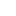喜欢 | 1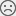不喜欢 | 0

JAVA工程师交流群 +加入

WEB/H5前端交流群 +加入•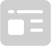资料索取
•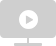答疑解惑
•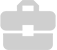技术交流
•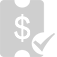职业测评
•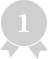面试技巧
•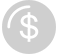高薪秘籍
Python30天热搜词 更多>>
X